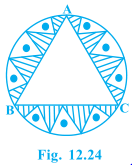• +91 9971497814
• info@interviewmaterial.com

# Areas Related to Circles Ex-12.3 Interview Questions Answers

### Related Subjects

Question 1 :

Find the area of theshaded region in Fig. 12.19, if PQ = 24 cm, PR = 7 cm and O is the centre ofthe circle.

Answer 1 :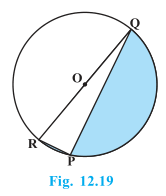Solution:

Here, P is in the semi-circle and so,

P = 90°

So, it can be concluded that QR is hypotenuseof the circle and is equal to the diameter of the circle.

QR = D

Using Pythagorean theorem,

QR= PR2+PQ2

Or, QR= 72+242

QR= 25 cm = Diameter

Hence, the radius of the circle = 25/2 cm

Now, the area of the semicircle = (πR2)/2

= (22/7)×(25/2)×(25/2)/2 cm2

= 13750/56 cm= 245.54 cm2

Also, area of the ΔPQR = ½×PR×PQ

=(½)×7×24 cm2

= 84 cm2

Hence, the area of the shaded region = 245.54cm2-84 cm2

= 161.54 cm2

Question 2 :

Find the area of theshaded region in Fig. 12.20, if radii of the two concentric circles with centreO are 7 cm and 14 cm respectively and AOC = 40°.

Answer 2 :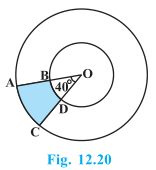Given,

Angle made by sector = 40°,

Radius the inner circle = r = 7 cm, and

Radius of the outer circle = R = 14 cm

We know,

Area of the sector = (θ/360°)×πr2

So, Area of OAC = (40°/360°)×πrcm2

= 68.44 cm2

Area of the sector OBD = (40°/360°)×πrcm2

= (1/9)×(22/7)×7= 17.11 cm2

Now, area of the shaded region ABDC = Area ofOAC – Area of the OBD

= 68.44 cm2 – 17.11 cm=51.33 cm2

Question 3 :

Find the area of theshaded region in Fig. 12.21, if ABCD is a square of side 14 cm and APD and BPCare semicircles.

Answer 3 :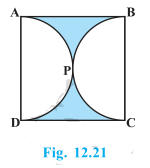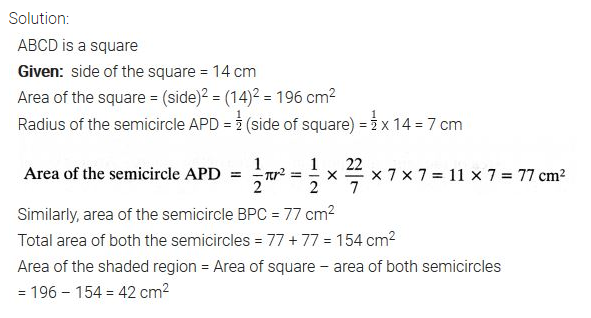Question 4 :

Find the area of theshaded region in Fig. 12.22, where a circular arc of radius 6 cm has been drawnwith vertex O of an equilateral triangle OAB of side 12 cm as centre.

Answer 4 :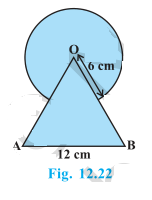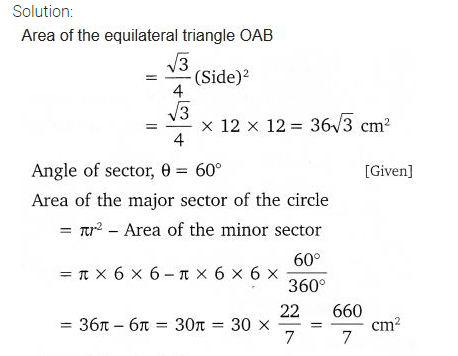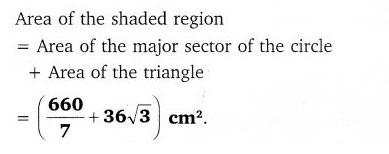Question 5 : From each corner of a square of side 4 cm aquadrant of a circle of radius 1 cm is cut and also a circle of diameter 2 cmis cut as shown in Fig. 12.23. Find the area of the remaining portion of thesquare.

Side of the square = 4 cm

Radius of the circle = 1 cm

Four quadrant of a circle are cut from cornerand one circle of radius are cut from middle.

Area of square = (side)2= 4=16 cm2

Area of the quadrant = (πR2)/4 cm2 =(22/7)×(12)/4 = 11/14 cm2

Total area of the 4 quadrants = 4 ×(11/14) cm2 =22/7 cm2

Area of the circle = πRcm2 =(22/7×12) = 22/7 cm2

Area of the shaded region = Area of square –(Area of the 4 quadrants + Area of the circle)

= 16 cm2-(22/7) cm2+(22/7)cm2

= 68/7 cm2

Question 6 : In a circular table cover of radius 32 cm, adesign is formed leaving an equilateral triangle ABC in the middle as shown inFig. 12.24.